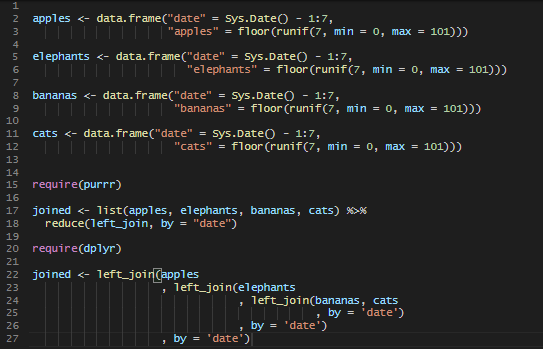# Multiple left joins in RHere is a quick and easy way to perform multiple left joins in R with multiple data frames.

Here are 4 data frames that I would like to join by the column date.

```apples <- data.frame("date" = Sys.Date() - 1:7,
"apples" = floor(runif(7, min = 0, max = 101)))

elephants <- data.frame("date" = Sys.Date() - 1:7,
"elephants" = floor(runif(7, min = 0, max = 101)))

bananas <- data.frame("date" = Sys.Date() - 1:7,
"bananas" = floor(runif(7, min = 0, max = 101)))

cats <- data.frame("date" = Sys.Date() - 1:7,
"cats" = floor(runif(7, min = 0, max = 101)))```

The fastest and easiest way to perform multiple left joins in R is by using reduce function from purrr package and, of course, left_join from dplyr.

```require(purrr)
require(dplyr)

joined <- list(apples, elephants, bananas, cats) %>%
reduce(left_join, by = "date")```

If you have to combine only a few data sets, then other solutions may be nested left_join functions from the dplyr package. For more than 3 data frames, that is quite a struggle.

```require(dplyr)

joined <- left_join(apples
, left_join(elephants
, left_join(bananas, cats
, by = 'date')
, by = 'date')
, by = 'date')```

If you want to know how to reflow your code or other useful RStudio tips and tricks, take a look at this post.